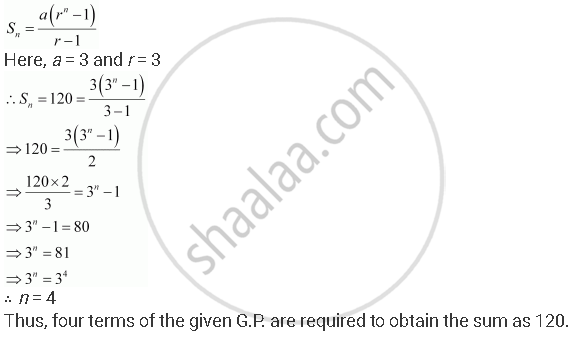CBSE (Arts) Class 11CBSE
Share
Notifications

View all notifications
Books Shortlist
Your shortlist is empty

# How Many Terms of G.P. 3, 32, 33, … Are Needed to Give the Sum 120? - CBSE (Arts) Class 11 - Mathematics

Login
Create free account

Forgot password?
ConceptGeometric Progression (G. P.)

#### Question

How many terms of G.P. 3, 32, 33, … are needed to give the sum 120?

#### Solution

The given G.P. is 3, 32, 33, …

Let n terms of this G.P. be required to obtain the sum as 120.Is there an error in this question or solution?

#### APPEARS IN

NCERT Solution for Mathematics Textbook for Class 11 (2018 to Current)
Chapter 9: Sequences and Series
Q: 13 | Page no. 192
Solution How Many Terms of G.P. 3, 32, 33, … Are Needed to Give the Sum 120? Concept: Geometric Progression (G. P.).
S